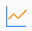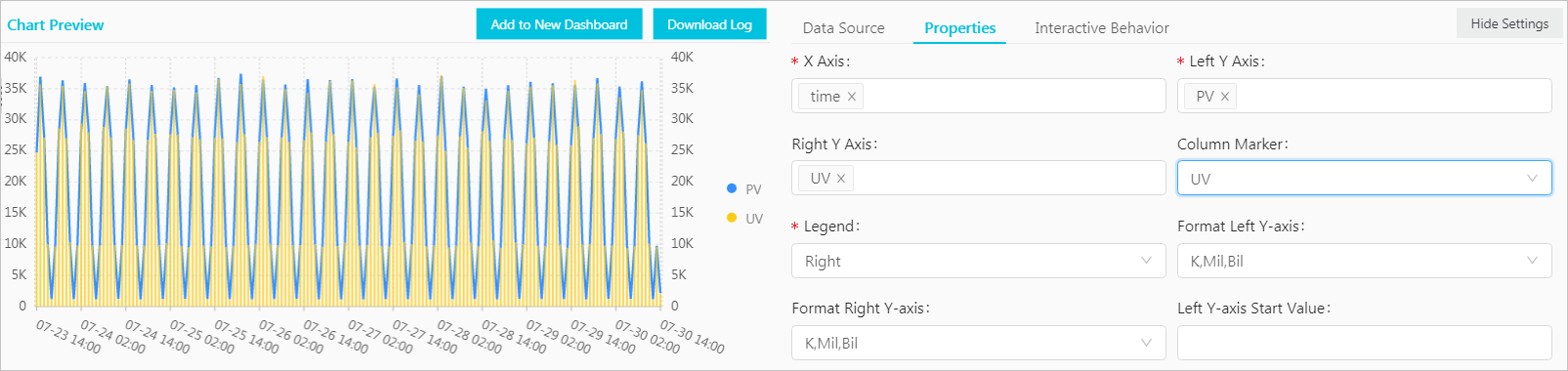This topic describes how to view query results in a line chart.

## Background information

A line chart is used to analyze the value changes of a categorical variable over a continuous time range. In most cases, the analysis is based on a specified time range. You can intuitively view value trends. You can use a line chart to analyze the following change characteristics of variable values over a specified time range:
• Increment or decrement
• Increment or decrement rate
• Increment or decrement pattern, such as, periodicity
• Peak value and bottom value

Line charts are the optimal choice for you to analyze value changes over a time range. You can also use a line chart to analyze the value changes of multiple categorical variables in multiple lines over the same time range. This allows you to analyze the relationship between the categorical variables. For example, the values of multiple categorical variables can show positive, negative, or inverse trends.

Each line chart consists of the following elements:
• X-axis
• Left Y-axis
• Right Y-axis (optional)
• Data point
• Line of trend changes
• Legend

## Procedure

1. Log on to the Log Service console.
2. In the Projects section, click the name of the project that you want to manage.
3. Choose Log Storage > Logstores and click the Logstore that you want to manage.
4. Enter a query statement in the search box, select a time range, and then click Search & Analyze.
5. On the Graph tab, click theicon.
6. On the Properties tab, configure the properties of the line chart.
Note In a line chart, each line must contain more than two data points. Otherwise, the data trend cannot be generated. We recommend that you select no more than five lines for a line chart.
Parameter Description
X Axis The categorical variable. In most cases, time series is selected.
Left Y Axis The value of the categorical variable. You can select one or more fields for the left Y-axis.
Right Y Axis The categorical variable. You can select one or more fields for the right Y-axis. The layer of the right Y-axis is higher than that of the left Y-axis.
Column Marker The field based on which the column chart is displayed. You must select this field from the fields that you select for Right Y Axis or Left Y Axis.
Legend The position of the legend in the chart. Valid values: Top, Bottom, Left, and Right.
Format Left Y-axis The format in which data on the left Y-axis and right Y-axis is displayed.
Format Right Y-axis
Left Y-axis Minimum Value The minimum value for the left Y-axis and right Y-axis.
Left Y-axis Minimum Value
Left Y-axis Maximum Value The maximum value for the left Y-axis and right Y-axis.
Right Y-axis Maximum Value
Line Type The type of line that is displayed in the line chart. Valid values: Straight Line and Curve.
Show Markers If you turn on Show Markers, the data points are displayed.
Metric Filter If you turn on Metric Filter, you can filter metrics to view specific types of data.
Legend Width The width of the legend.
X-axis Scale Density The scale density of the X-axis. Valid values: 3 to 30.
Scatter Column The Y-axis column where dots are located.
Scatter Numeric Column The value on the dot column.
Scatter Size The value range of the dots.
Anomaly Point Column The column whose anomaly points you want to view. You can configure the Anomaly Point Lower Limit and Anomaly Point Upper Limit parameters for a column.
• Anomaly Point Lower Limit: Values that are less than the lower limit are highlighted in red.
• Anomaly Point Upper Limit: Values that exceed the upper limit are highlighted in red.
Upper Limit Column The area that is formed based on the values.
Lower Limit Column
Time Series The series of data points that are listed in chronological order.
Time Format The time format of the time series.
Margin The distance between the axis and the borders of the chart. The parameters include Top Margin, Bottom Margin, Left Margin, and Right Margin.

## Example of a simple line chart

To query the number of page views (PVs) for the IP address `10.0.0.0` in the last 24 hours, execute the following query statement:

``````remote_addr: 10.0.0.0 | select date_format(date_trunc('hour', __time__), '%m-%d %H:%i')
as time, count(1) as PV group by time order by time limit 1000``````

Select `time` for X Axis, `PV` for Left Y Axis, and Bottom for Legend. Adjust the margins based on your business requirements.## Example of a dual-axis line chart

To query the numbers of PVs and unique visitors (UVs) in the last 24 hours, execute the following query statement:

``* | select date_format(date_trunc('hour', __time__), '%m-%d %H:%i') as time, count(1) as PV, approx_distinct(remote_addr) as UV group by time order by time limit 1000``

Select `time` for X Axis, `PV` for Left Y Axis, `UV` for Right Y Axis, and `UV` for Column Marker.